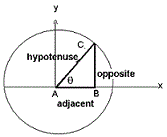# Trigonometry Calculator

The trigonometric functions have been defined using the unit circle. The degree of an angle measured counter-clock-wise from the x-axis along an arc of the circle.

 Sine(Sin) Cosine(Cos) Tangent(Tan) Cotangent(Cot) Secant(Sec) Cosecant(Cosec)
 Arc Sine(ASin) Arc Cosine(ACos) Arc Tangent(ATan) Arc Cotangent(ACot) Arc Secant(ASec) Arc Cosecant(ACosec)

#### Degrees

 Sine(Sin) Cosine(Cos) Tangent(Tan) Cotangent(Cot) Secant(Sec) Cosecant(Cosec)
 Arc Sine(ASin) Arc Cosine(ACos) Arc Tangent(ATan) Arc Cotangent(ACot) Arc Secant(ASec) Arc Cosecant(ACosec)

The sin(θ) is the vertical component , the cos(θ) is the horizontal coordinate of the arc endpoint and the ratio of sin(θ) / cos(θ) is defined as tan(θ). Since the trigonometric functions are periodic with period 360 degree or say 2 π therefore :

func(θ) = func( 2 π r n + θ)

where func is a trigonometric function and n is an integer.

Sin(q) = Opposite / Hypotenuse